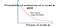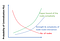# Universe is a p2p network

[Disclaimer: There are several assumptions in this article and some claims might be wrong. Please correct me.]

I somewhat believe that the universe is essentially a p2p network and the nature of p2p network (node type, node-node interaction, no. of nodes, centralization, etc.) changes with the scale. Once I even tweeted wondering if supernatural entities are decentralized and maybe exist as p2p networks. That’s some crazy idea. https://twitter.com/GajenJung/status/922560091299049472

## Few examples of how p2p networks change with the scale

At its core (limited by our understanding of particle physics), universe is a p2p network of quarks and leptons. The quarks tend to interact with each other and form more complex nodes i.e. protons, atoms and so on. At the extreme end, universe is a p2p network of galaxies interacting with each other via gravitational and electromagnetic forces.

# What is a p2p network?

A p2p network is a group of similar elements (let’s say nodes) that interact with each other and can be regarded to exist and function as a single entity in some conceptual level. Three fundamental characteristics of a p2p network are:

Hierarchy of p2p networks

A p2p network always exists within an environment. The environment itself is a higher-level p2p network or a group of p2p networks (e.g. bitcoin network exists inside the human ecosystem) or one of its node (e.g. solar system exists inside the Milky Way galaxy, here Milky way is a node in the network of galaxies). The environment affects all the three fundamental characteristics of a p2p network and hence other secondary properties. Since the effects of environment is already factored into these characteristics, I will neglect the environment from here on. [However, in practice, the p2p network will also affect the environment, i.e. there is a two-way interaction which is always happening.]

## What does it mean to be decentralized?

A group (e.g. p2p network) is decentralized if its constituent elements have a similar amount of power to control the existence and functioning of the group. Decentralization of a group can be thought as a lack of order in the power of its constituent elements to act as a group i.e. power structure of the group and the ‘power’ is dependent on the type of p2p network.

Few examples of p2p network and ‘power’ of nodes:

# Using entropy to measure decentralization

Decentralization of a group is the lack of order in the power of its members, so the degree of decentralization would be equivalent to the measure of disorder or randomness, similar to the concept of entropy https://en.wikipedia.org/wiki/Entropy. Entropy is a measure of the number of different ways that a set of objects can be arranged https://link.springer.com/article/10.1186/1936-6434-6-30. In statistical mechanics, entropy is the measure of possible number of micro-states for a macro-state. For a p2p network, the equivalent would be a metric proportional to the number of possible configurations of the nodes to form the network. In information theory, entropy is the average information carried by a symbol in a system i.e. group of symbols.

Here on, I will use entropy as a metric to quantify decentralization and I will mainly use the information-theoretic perspective.

[I think entropy from the perspective of classical mechanics could have been used where the entropy is defined as measure that is proportional to the number of micro-states for a macro-state. For a p2p network, the entropy would be a metric proportional to the number of possible configurations of the nodes to form the network.]

## Entropy in information theory

Here, I will briefly explain information-theoretic view of entropy and relate that to the decentralization of a p2p network. According to Shannon’s information theory, entropy is the average information content of an event in a system. For example, for a language with a set of symbols, it’s entropy is the average information contained in a symbol.

Entropy H = ∑p(x).I(x) = -∑p(x).log(p(x))

where:

x is a symbol (node for a p2p network) representing some information, p(x) is the probability of occurrence of x and I(x) = -log(p(x)) is the information content in x (the power of the node or symbol x). The rarer the x is (i.e. p(x) is small), greater the information content (i.e I(x) is large).

This provides an important insight: the probability of existence/occurrence of a node decreases with increase in its power over the network.The probability of existence of a node decreases with its power over the network.

Examples:

3. Power = mass. In the figure below, the number of planets (equivalent to p(x)) decreases with their masses (their power).

# Entropy in a p2p network

Let’s assume that the measure of decentralization in a p2p network can be formulated as: H + N-N

where:

H is the entropy assuming there is no node-node interactions. It is proportional to the number of possible configurations of the nodes to form the network.

N-N is the effects of node-node interaction (complexity, strength, etc.). For example, in our solar system this term is affected by the distance (via gravitational force) and in the Bitcoin network, it will be affected by geography (e.g. power outage), political beliefs of the node owners, incentives of mining pools, etc.

# Probability of centralization P(c)Dependence of the probability of centralization P(c) on the characteristics of a p2p network.

## 1. Probability of centralization P(c) increases with the increase in the upper bound of node complexity

Let’s represent a p2p network by a set of realizations of random variable X (each unique x represent a possible node) and define following quantities.

a) Size of the Node Space (NS): the no. of theoretically possible states a node can occupy. This quantity is limited by the representation power of X (e.g. its dimensionality)

b) Size of the Occupied Node Space (ONS): the no. of nodes that actually occupy the node space in practice to form a network. ONS is generally a small subset of NS.

c) Effective support: ONS/NS

When the probability of existence of the nodes p(x) is uniform (See (a) in the figure below), the effective support of p(x) is maximum, max(p(x)) is minimum, and entropy is maximum; a state of complete decentralization. Now, let’s increase the upper bound of node complexity (similar to increasing the dimensionality of X). This exponentially increases the number of possible states a node can theoretically occupy i.e. there is increase in the size of node space NS. However, the no. of nodes that can occupy the node space in practice to form a network ONS do not increase in the same rate. This means the effective support of p(x) decreases and it becomes narrower in shape, thereby increasing the certainty or decreasing the entropy, which means, there is increase in centralization. Another way of thinking this is: when the node space increases, the actual nodes occupy only a manifold of the node hyperspace. This phenomena is related to the curse of dimensionality. https://en.wikipedia.org/wiki/Curse_of_dimensionality.

ExamplesProbability of centralization P(c) increases with the increase in node complexity.

## 2. Probability of centralization P(c) decreases with the increase in no. of nodes

When the no. of nodes in a network increases, the effective support of p(x)(the ratio between the no. of actual nodes in existence and the total no. of possible nodes) increases, which in turn increases the entropy or decentralization i.e. the probability of centralization P(c) decreases.

## 3. Probability of centralization P(c) increases with the increase in strength and complexity of node-node interaction

Centralization is a process when nodes in a network tend to interact with each other to create an order in the power of the nodes (e.g. forming groups or sub-networks). This increase in the order decreases the entropy and decentralization. Increase in the strength and complexity of the node-node interactions will allow the nodes to communicate and interact with each other more efficiently, thereby helping the process of centralization.

Examples

# Evolutionary force vs. Entropic force

It seems there are two opposing forces in the universe: 1) Entropic force (from 2nd law of Thermodynamics) which tend to increase the entropy and 2) Evolutionary force which tend to decrease the entropy by creating an order.

[Note: It seems this idea is not as novel as I thought and is actually pretty old and quite controversial. https://en.wikipedia.org/wiki/Entropy_and_life]

[There are few theories suggesting intelligence is equivalent to the reduction in entropy https://wellbredinsolence.wordpress.com/2010/05/13/is-entropy-the-secret-to-intelligence/, https://www.bbc.com/news/science-environment-22261742]

Examples of the increase in order with evolution

## Is Universe expanding to increase its entropy so that it can balance the evolutionary force?

From a p2p perspective of the universe, one way of increasing the entropy (and decentralization) is to decrease the strength and complexity of the node-node interaction. Since out of 4 fundamental forces, only gravitational and electromagnetic forces have infinite ranges and both of them decrease quadratically with the distance, a good way to decrease the node-node interaction is to increase the distance between the nodes. Maybe the universe is doing the same by expanding itself? :) [The expansion of the universe doesn’t actually increase its entropy, however the expansion allows it be in a state that can attain more entropy (more choices)].

[BTW there are few theories that claim gravity itself is an entropic force https://arxiv.org/pdf/1001.0785.pdf]

## How do we design our own p2p networks learning from natural p2p networks?

next medium article

## Using entropy for the comparison of PoW vs. POS vs. DPOS ?

next medium article

Scientist